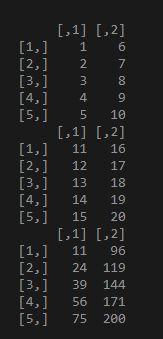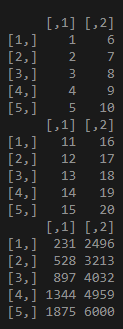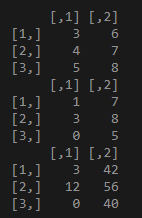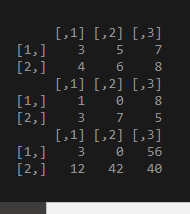Elementwise Matrix Multiplication in R

• Last Updated : 26 Mar, 2021

In a matrix, as we know rows are the ones that run horizontally and columns are the ones that run vertically. In this article, we are going to perform element-wise matrix multiplication in R programming.

Approach

• Create a matrix
• Multiply two matrices
• Verify the result.

Element-wise multiplication using “*” operator:

Syntax: matrix1*matrix*2…..matrix n

Example 1:

This code shows the element-wise multiplication of two matrices data1 and data2, Data comprises 5 rows and 2 columns:

R

 # Creating matrices 10 elements each using# range operator ":"data1 <- matrix(1:10, nrow = 5) print(data1)  data2 <- matrix(11:20, nrow = 5) print(data2)  # perform element wise multiplicationprint(data1*data2)

Output:Example 2:

This code for multiplication of multiple matrices data1,data2,data3. All data comprises 5 rows created using the range operator.

R

 # Creating matrices 10 elements each # using range operator ":"data1 <- matrix(1:10, nrow = 5) print(data1)  data2 <- matrix(11:20, nrow = 5) print(data2)  data3 <- matrix(21:30, nrow = 5)  # perform element wise multiplicationprint(data1*data2*data3)

Output:Example 3:

This code shows the matrix is created using vectors. And matrix multiplication is done.

R

 # vector aa = c(3, 4, 5, 6, 7, 8)  # vector bb=c(1, 3, 0, 7, 8, 5)  # Creating matrices using vectordata1 <- matrix(a, nrow = 3) print(data1)  data2 <- matrix(b, nrow = 3) print(data2)  print(data1*data2)

Output:Example 4:

An example that shows multiplication column arrangement and matrices data1 and data2 and multiplied. Column wise we are going to perform matrix multiplication data1 and data2 comprises 3 columns and elements are created using vector.

R

 # vector aa = c(3, 4, 5, 6, 7, 8)  # vector bb = c(1, 3, 0, 7, 8, 5)  # Creating matrices using vectordata1 <- matrix(a, ncol = 3) print(data1)  data2 <- matrix(b, ncol = 3) print(data2)  print(data1*data2)

Output:My Personal Notes arrow_drop_up